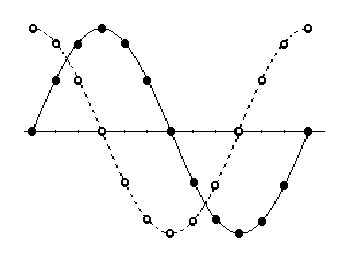Daqarta
Data AcQuisition And Real-Time Analysis
Scope - Spectrum - Spectrogram - Signal Generator
Software for WindowsThe following is from the Daqarta Help system:

# Sine Wave Phase

Phase is the measure of the starting point of one sinusoid relative to another. In the sine wave clock example the reference starting point was the 9 o'clock position, so any wave that starts there is "in phase" with the reference wave. If a wave starts anywhere else, its angle relative to this reference is its "phase angle". A wave that starts at 6 o'clock (cosine) is thus at an angle of 90 degrees relative to the reference sine. The cosine (open dots) reaches 12 o'clock just as the sine (solid dots) hits 9 o'clock to start a new cycle:In mathematics the 3 o'clock position is always used as the starting point, and everything runs counter-clockwise. Apart from a sign change, the results are the same.

If two identical sinusoids are added together, the result is a sinusoid with twice the amplitude. If the two waves are shifted so that they are 180 degrees out of phase, then the positive portions of one wave will cancel the negative portions of the other and the sum will be zero. At intermediate phase angles, the sum can vary over the range from 0 to 200%. At 120 or 240 degrees, the sum will be the same magnitude as either of the original values, but at an intermediate phase.GO: